Question

A student dissolved 0.44g of copper wire and followed all steps described in the copper cycle experiment to obtain solid copper. After performing the series of reactions a student weighed a total mass of 24.61g on the scale. This mass included the filter paper mass of 0.23g and the watch glass of 24.19g. Calculate the % recovery for this experiment. %recovery= final mass of copper grams divided by initial mass of copper grams

Initial mass of Copper = 0.44 g

Mass of filter paper = 0.23 g

Mass of watch glass = 24.19 g

Mass of filter paper and watch glass = 0.23 + 24.19 = 24.42 g

Total mass = 24.61 g

Mass of copper recovered = 24.61 – 24.42 = 0.19 g

% recovery = Mass of copper recovered /Initial mass of Copper *100

% recovery = 0.19 g / 0.44 g*100 = 43.18182 % = 43.18 %

#### Earn Coins

Coins can be redeemed for fabulous gifts.

Similar Homework Help Questions
• ### THE COPPER CYCLE: A Series of Reactions Experimental Data From Experimental Procedure - Part A 1....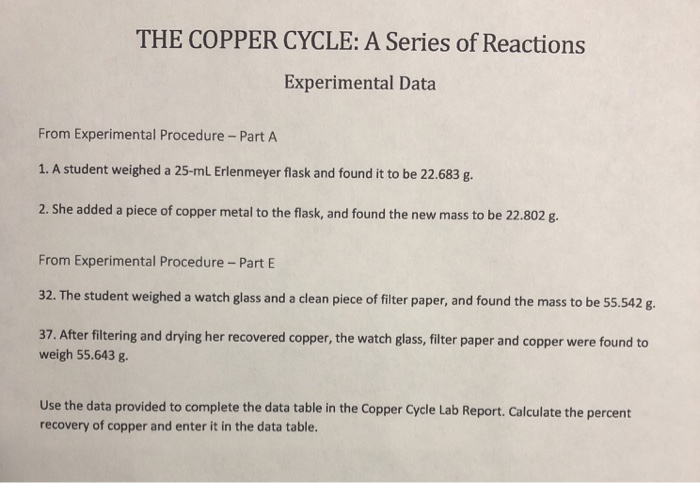THE COPPER CYCLE: A Series of Reactions Experimental Data From Experimental Procedure - Part A 1. A student weighed a 25-ml Erlenmeyer flask and found it to be 22.683 g. 2. She added a piece of copper metal to the flask, and found the new mass to be 22.802 g, From Experimental Procedure - Part E 32. The student weighed a watch glass and a clean piece of filter paper, and found the mass to be 55.542 g. 37. After...

• ### A student dissolved a piece of copper wire, then converted it to Cuo, filtered the Cuo...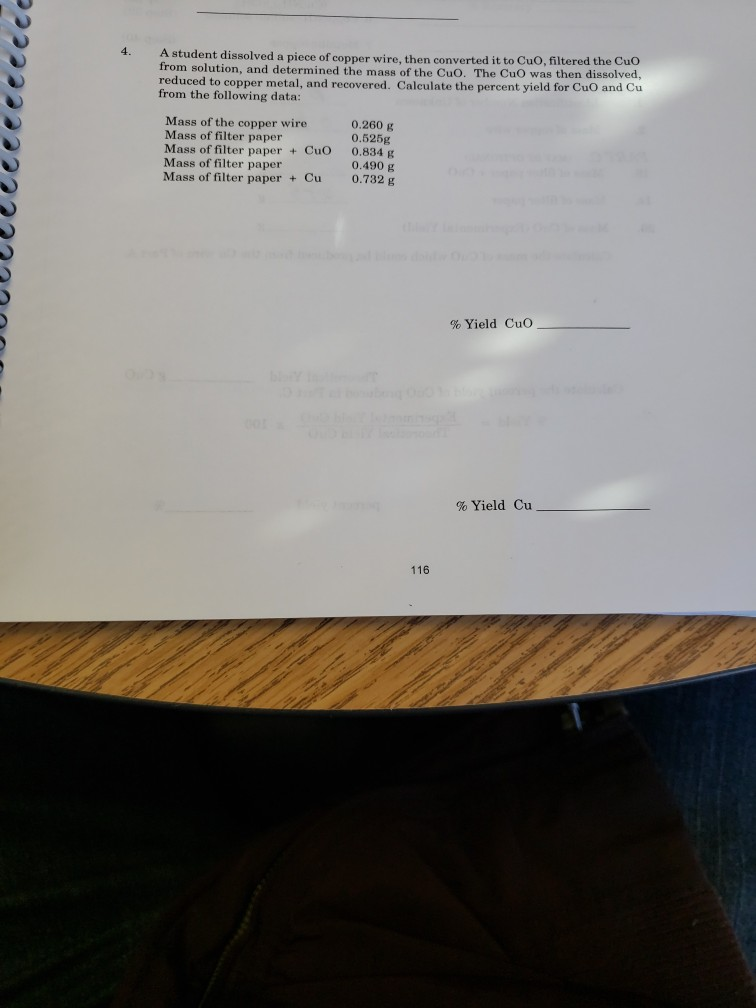A student dissolved a piece of copper wire, then converted it to Cuo, filtered the Cuo from solution, and determined the mass of the Cuo. The CuO was then dissolved, reduced to copper metal, and recovered. Calculate the percent yield for CuO and Cu from the following data: UULUcceeeeeeeee Mass of the copper wire Mass of filter paper Mass of filter paper + CuO Mass of filter paper Mass of filter paper + Cu 0.260 g 0.5258 0.834 g 0.490...

• ### Heat lamp or drying oven Hydrated copper chloride Distilled water Aluminum wire 30-ml beaker Glass rod...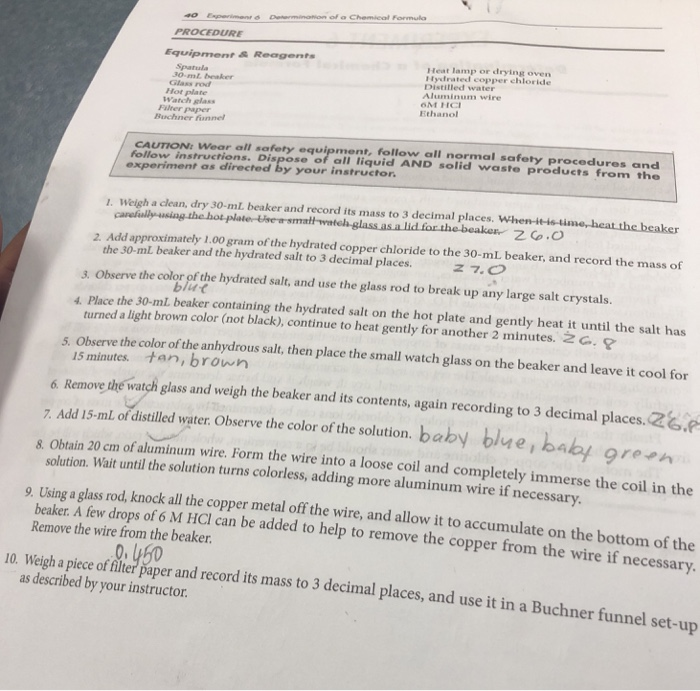Heat lamp or drying oven Hydrated copper chloride Distilled water Aluminum wire 30-ml beaker Glass rod Watch glass Buchner fiannel Hot plate Ethanol Füter paper CAUTION: Wear all safety equipment, follow all normal safety procedures and experiment as directed by your instructor follow instructions. Dispose of all liquid AND solid waste products from the 1. Weigh a clean, dry 30-mL beaker and record its mass to 3 decimal places. When-it-is-time,heat the beaker 2. Add approximately 1.00 gram of the hydrated...

• ### Determine the percent yield of copper. 3. What is the theoretical yield of copper produced by...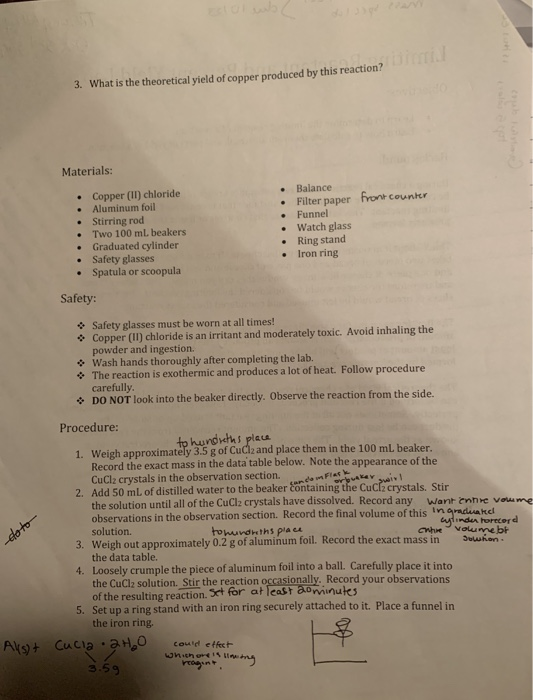Determine the percent yield of copper. 3. What is the theoretical yield of copper produced by this reaction? Materials: Copper (II) chloride • Aluminum foil • Stirring rod • Two 100 ml beakers • Graduated cylinder • Safety glasses • Spatula or scoopula • Balance Filter paper front counter Funnel • Watch glass Ring stand • Iron ring Safety: Safety glasses must be worn at all times! Copper (II) chloride is an irritant and moderately toxic. Avoid inhaling the powder...

• ### 2. Define the following: a. acid-base reaction b. displacement reaction c. oxidation-reduction reaction d. weak electrolyte...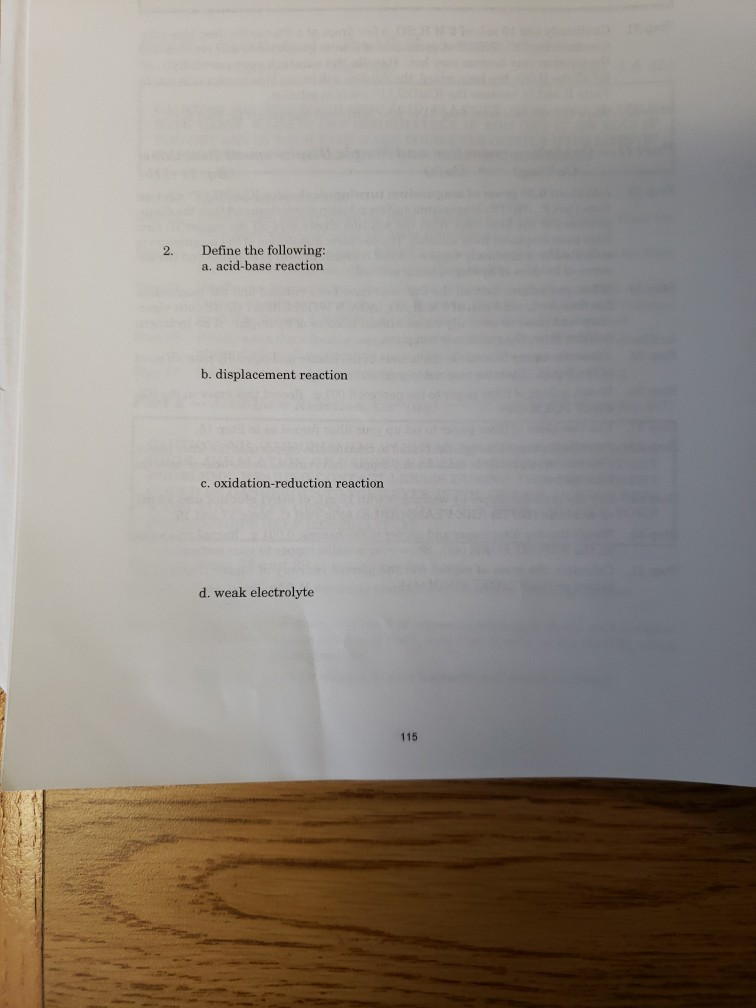2. Define the following: a. acid-base reaction b. displacement reaction c. oxidation-reduction reaction d. weak electrolyte 115 A Sequence of Chemical Reactions 3. PPPPPPPPPP Identify the following equations as single or double displacement, redox, acid-base, combination, or decompostion reactions. Some may fit in more than one category. a. Cu(s) + 4HNO, (aq) → Cu(NO),(aq) + 2NO, + 2H,0 Mg(s) + H.SO(aq) - MgSo. (aq) + H (6) CuO(s) + HCl(aq) → Cuci (aq) + H,000 d. Cu(NO),(aq) + 2NaOH(aq ->...

• ### Lab procedure: This laboratory exercise involves the use of dilute sulfuric acid (H2SO4) to leach...

Lab procedure: This laboratory exercise involves the use of dilute sulfuric acid (H2SO4) to leach copper from a synthetic copper-containing ore, malachite. This technique is known in the mining industry as solution mining. The soluble copper is then filtered to remove unreacted ore (the residue) from the filtrate containing the copper+2 ion. The copper in the filtrate is then treated with metallic magnesium to precipitate the copper as the familiar orange-brown metal. This copper is dried and weighed. From these...

• ### Make sure the reaction table described above is completed in your notebook.

Prelab questions:1. Make sure the reaction table described above is completed in your notebook.2. Two benzaldehyde molecules combine with one acetone molecule in this aldol reaction. If someone uses 5.0 mL of benzaldehyde and 2.5 mL of acetone to try to improve the yield of product, is this the correct ratio of molecules so that there is no excess? Why or why not?The Aldol Reaction: Synthesis of DibenzalacetonePurpose: Introduce the student to the hands-on chemistry of carbonyl condensations.Warning: Dispose of...

• ### Observations Record observations including appearance of solution (clear, cloudy), color(s) of liquid and s...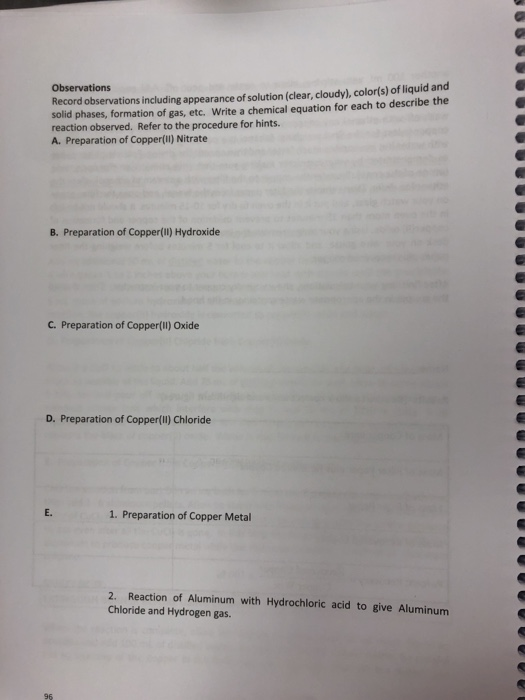Observations Record observations including appearance of solution (clear, cloudy), color(s) of liquid and solid phases, formation of gas, etc. Write a chemical equation for each to describe the reaction observed. Refer to the procedure for hints. A. Preparation of Copper(ll) Nitrate B. Preparation of Copper(1) Hydroxide C. Preparation of Copper(ll) Oxide D. Preparation of Copper(II) Chloride E. 1. Preparation of Copper Metal 2. Reaction of Aluminum with Hydrochloric acid to give Aluminum Chloride and Hydrogen gas. EXPERIMENT SA THE MANY...

• ### Objective Find the ratio of moles of reactant to moles of a product for the reaction...

Objective Find the ratio of moles of reactant to moles of a product for the reaction below. Compare mass and mole amounts of reactants to determine the amount of products that can be/are made. Background Chemical reactions are represented by balanced chemical equations. Proper interpretation of an equation provides a great deal of information about the reaction it represents and about the substances involved in the reaction. For example, the coefficients in a balanced equation indicate the number of moles...

• ### Experiment 8 Conservation of Mass kussion: action: 170 Mole wt. g/mol Aqual the combined masses of...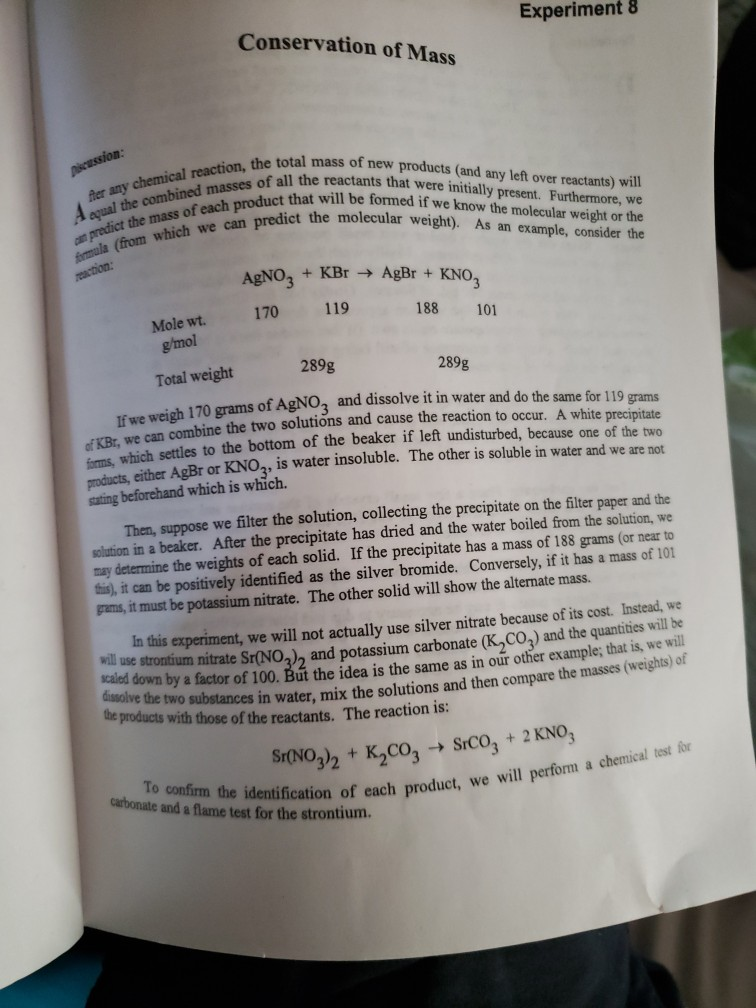Experiment 8 Conservation of Mass kussion: action: 170 Mole wt. g/mol Aqual the combined masses of all the reactants that were initially present. Furthermore, we fter any chemical reaction, the total mass of new products and any left over reactants) will a predict the mass of each product that will be formed if we know the molecular weight or the formula (from which we can predict the molecular weight). As an example, consider the dissolve the two substances in water,...